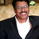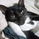# Nifty is in right track towards 10620_10700 minimum

NSE:NIFTY   Nifty 50 Index
623 views
Meanwhile there will be some corrections, those levels can be checked on a weekly basis,
But over all trend is a strong bullish one, hopes that the channel also shows a confluence resistance around 10700 level.

By this time many of you are familiar with the attached chart and hope you are confident about the degree of main trend. Definitely there are counter trend in various degree, and sometime we get misled with the internal waves. This time I am attaching something different, about the Fibonacci resistance/supports, which will also be a continuation of the subject we said here about time & price square'. but I cannot cover it all in one go. so please be patients and learn little by little.

There are four basic methods to find a resistance/ supports with Fibonacci ratios using retracements, extensions, expansion and price projections. Out of which retracement is familiar to most of us. An expansion is like extending the same price line from a correction point. Here I am showing the next possible minimum target in nifty' with PROJECTION of wave 1 and EXTENSION of wave 2. A confluence of many retracement together have more probabilities to see target/ support, which we will discuss further. I will be on vacation for three weeks, still I would like to be here on my leisure, will come up with more. But remember these are all only probability & not surety. Following is Just for study only.

The following chart showing a projection of wave 1 of from the wave 3 start. It shows the 127% of wave 1 will have a target around 10619.

And an extension just opposite to retrace, look here the wave 2 extension met at 1.786 times is 9813 achieved, next gives us a 2.272 is targeting10335 and a 2.618 time wave 2 is coming around 10700.

The properties of this series were made famous by the Italian mathematician Leonardo de Pisa. The Fibonacci number series starts with 0 and 1 and goes out to infinity, with the next number in the series being derived by adding the prior two. For example, 55 + 89 = 144, 89 + 144 = 233, 144 + 233 = 377, and so on (see the following number series): 0, 1, 1, 2, 3, 5, 8, 13, 21, 34, 55, 89, 144, 233, 377, 610, 987……..

What is most fascinating about this number series is that there is a constant found within the series as it progresses toward infinity. In the relationship between the numbers in the series, you will find that the ratio is 1.618, or what is called the Golden Ratio, Golden Mean, or Golden or Divine Proportion. (For example, 55 x 1.618 = 89, and 144 is 1.618 times 89.) Take any two consecutive numbers in the series after you get beyond the first few and you will find the Golden Ratio.

We can also find the ratio in nature. It can be seen in flowers, the nautilus' shell, ammonite fossils, and many other places.

The main ratios useful in our everyday analysis are 0.382, 0.50, 0.618, 0.786, 1.00, 1.272, and 1.618. we can also include 0.236, 2.618, and 4.236 they are all related mathematically.

eg: 1.0-0.618 = 0.382
0.618x0.618 = 0.382
1.0/2 = 0.50
Square' root of 0.618 = 0.786
0.618 is the reciprocal of 1.618
Square' root of 1.618 = 1.272
0.618x0.382 = 0.236
1.618x1.618 = 2.618
2.618x1.618 = 4.236

We can also find our trading setups or finding probabilities by applying the main Fibonacci ratios on the price axis of the market. There are four basic methods to find a resistance/ supports with Fibonacci ratios using
retracements, extensions, expansion and price projections. We will study this to combine with our time & price squaring.

There are many Web sites that are devoted to this number series and its properties. Just type the word Fibonacci into your favorite search engine and you’ll be amazed at the power of information that exists on this subject. It will be useful to you in market studies if you get it through.
See you all.
Comment: welcome you all
Comment: Good morning to all
Comment: Q&A
Even though it is hypothetical ,
a simple charting technique says,
it can attain that target 10700 at
maximum by the end of November,
with the current pace.ravi923615
@ravi923615,
10700 is just a minimum target we assumed, a 127% of wave1, can attain is short time,

While there is no minimum time constraint for Wave 3, its absolute maximum time limit is 7 times Wave 1. That means soon it can find a top or go months more, and why we want to stop it. Let it go to 200 to 400% of wave 1, we should not ask time limit for wave 3. That should be our approach. Mind that we are talking about waves, and not about a swing movement. Both should have different view. If you are asking a time frame for a swing it is worth asking questions.

Wave 3 is usually faster, steeper, & sharper of all waves with formation with gaps in between because investors and traders are fully assertive of the trend and they want to buy at any levels especially when wave 3 crosses above the end of wave 1. Wave 3 is normally extend anywhere between 100% - 261.8% of wave 1 starting from the end of wave 2 can be of even 685% or 1109% of wave 1. The history says, we should not estimate the top of wave 3 and we should not book profit during the progress of wave 3, a trailing stop loss at every top is best approach for maximum profit. The trader who tries to chase wave 3 often get hoodwinked.Agreed. in between 10036 & 10628.35, here is another point also Crucial, 10315.35 (FiB Projection 1.13, in between 1.0 & 1.272.)

Also 1st Extended ABCD pattern.pankajvw
@pankajvw, The value equivalent to that nifty price may be apporx:25950,

a 100% Fibonacci projection is marked in blue labels, but the hypothesis shows, it has to move much more as per the classic Elliot rules.

A 127% projection (blue)of wave 1 = 29358 must reach minimum.
So assuming a value near to this.
But couldn’t find an ebullient value in wave 2 extension group (pink).
161.8% projection of wave is 29707
Wave extensions in green can go anything above 24777 ie: greater than 100%of wave